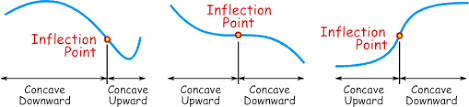# Cutter Output curve to mesh

Hello everyone,
Is it possible to convert the output curve of a vtkCutter class to a mesh? I wanted to compute curvatures but vtkCurvatures only takes a polydata mesh as input. I tried using vtkDelaunay2D but the mesh is irregular. And I tried to smooth the mesh using vtkWindowedSincPolyDataFilter but the filter does not give the result that I want. I also played with the number of iterations method. I would like to get the points from the curve where the curvature changes i.e. from convex to concave or concave to convex.

…maybe there’s some more clever way… one possibility is to fit analytically (superfast) a circle to the line locally and look at the cross product to its center to get the sign of the curvature:

``````import numpy as np
from vedo import *

shape = Spline([
[0.0, 0.0],
[1.0, 0.0],
[1.1, 4.0],
[3.0, 1.5],
],
res=100,
smooth=0,
).lineWidth(4)

points = shape.points()
fitpts=[]
circles=[]
curvs=[]
n=4
for i in range(shape.NPoints()-n):
pts = points[i:i+n]
cx, cy, R = fitCircle2D(pts)
z = np.cross(pts[-1]-pts, pts-[cx,cy,0])
curvs.append(np.sqrt(R))
if R<1:
fitpts.append([cx,cy])
circle = Circle([cx, cy], r=R).wireframe().color(int(z+1))
circles.append(circle)
curvs += [curvs[-1]]*n

show(shape, circles, Points(fitpts), axes=1)

print(type(shape.polydata()))
``````

check out the algorithm here.

I see that you use 3D Slicer. We have recently added measurements for markups. Curve measurements include curvature (we implemented a new VTK class for curve curvature computation). All you need to run vtkCutter output through a vtkStripper to order the random line segments into a continuous curve then set points of the resulting polydata as input to the curve node in Slicer - about 8-10 lines of Python code in total. Or, if you work in C++ then you can take the vtkCurveMeasurementCalculator class from Slicer (it does not have any Slicer dependency), and use it in your software.

Hello @lassoan,
Thank you for the reply! I did not see any difference in the image of the curve that I attached earlier with the vtkStripper class. Is there any way to get the scalars(curvatures) and determine the RAS coordinates of a point where the sign of the curvature is changed? I saved the obtained curvatures from the vtkCurvatures class in a vtkDoubleArray and iterated over the array and determined the possible points(RAS). Is it somehow possible to do the same thing with the vtkCurveMeasurementCalculator class?Best regards,
Anish

vtkCurvature is for surfaces, not curves. Instead, you can use vtkCurveMeasurementCalculator class in Slicer or similar VTK classes in VMTK, etc. I would assume there are also tons of computational geometry packages in Python that can compute curvature of curves.